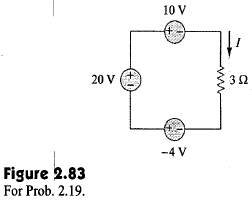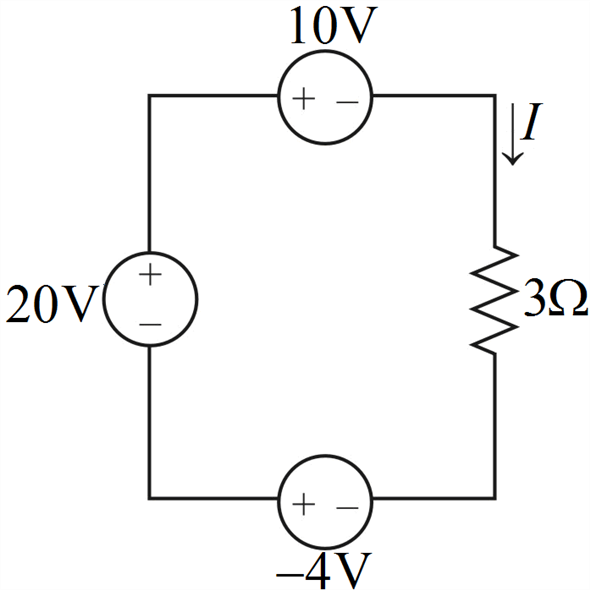Problem

# From the circuit in Fig. 2.83, find I,the power dissipated by the resistor, and the power...

From the circuit in Fig. 2.83, find I,the power dissipated by the resistor, and the power absorbed by each source.#### Step-by-Step Solution

Solution 1

The given circuit isBy applying Kirchhoff's voltage law to the above circuit, we obtain

\begin{aligned} &-20+10+3 I-(-4)=0 \\ &3 I=6 \\ &\therefore I=2 \mathrm{~A} \end{aligned}

Power dissipated by the resistor is

\begin{aligned} &p_{3 \Omega}=I^{2} \times 3 \\ &p_{3 \Omega}=(2)^{2} \times 3 \\ &\therefore p_{3 \Omega}=12 \mathrm{~W} \end{aligned}

Power absorbed by $$20 \mathrm{~V}$$ voltage source is,

$$p_{1}=20(-2)$$

Since for $$p_{1}, 2 \mathrm{~A}$$ current flows into the negative terminal of the source. $$\therefore \quad p_{1}=-40 \mathrm{~W} \quad$$ Supplied power

Power absorbed by $$10 \mathrm{~V}$$ voltage source is,

$$p_{2}=10 \times 2$$

Since for $$p_{2}, 2 \mathrm{~A}$$ current flows into the positive terminal of the source. $$\therefore p_{2}=20 \mathrm{~W}$$ Absorbed power

Power absorbed by $$-4 \mathrm{~V}$$ voltage source is,

$$p_{3}=(-4)(-2)$$

Since for $$p_{3}, 2 \mathrm{~A}$$ current flows into the negative terminal of the source.

$$\therefore p_{3}=8 \mathrm{~W}$$ Absorbed power